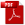Download as PDFA proof of Bertrand's postulate
Journal of the Indian Mathematical Society, XI, 1919, 181 – 182

1. Landau in his Handbuch, pp. 89 – 92, gives a proof of a theorem the truth of which was conjectured by Bertrand: namely that there is at least one prime $p$ such that $x \lt p\leq 2x$, if $x \geq 1$. Landau's proof is substantially the same as that given by Tschebyschef. The following is a much simpler one.

Let $\nu(x)$ denote the sum of the logarithms of all the primes not exceeding $x$ and let

\begin{equation} \Psi(x) = \nu(x) + \nu(x^{\frac{1}{2}}) + \nu(x^{\frac{1}{3}}) + \cdots, \end{equation}
\begin{equation} \log[x]! = \Psi(x) + \Psi(\half x) + \Psi(\myfrac{1}{3} x) + \cdots, \end{equation}
where $[x]$ denotes as usual the greatest integer in $x$.

From (1) we have

\begin{equation} \Psi(x) - 2\Psi (\sqrt{x}) = \nu(x) - \nu(x^{\frac{1}{2}}) + \nu(x^{\frac{1}{3}})- \cdots, \end{equation}
and from (2)
\begin{equation} \log[x]! - 2 \log[\half x]! = \Psi(x) - \Psi(\half x) + \Psi(\myfrac{1}{3} x)-\cdots. \end{equation}
Now remembering that $\nu(x)$ and $\Psi(x)$ are steadily increasing functions, we find from (3) and (4) that
\begin{equation} \Psi(x) - 2\Psi (\sqrt{x}) \leq \nu(x) \leq \Psi(x); \end{equation}
and
\begin{equation} \Psi(x) - \Psi (\half x) \leq \log [x]! - 2 \log [\half x]! \leq \Psi(x) - \Psi(\half x) + \Psi(\myfrac{1}{3}x). \end{equation}
But it is easy to see that
\begin{eqnarray} \log \Gamma(x) - 2 \log \Gamma (\half x + \half ) \leq \log [x]! - 2 \log [\half x]!\notag\\ \leq \log \Gamma(x+1) - 2 \log \Gamma (\half x + \half ). \end{eqnarray}
Now using Stirling's approximation we deduce from (7) that
\begin{equation} \log [x]! - 2\log[\half x]! \lt \myfrac{3}{4} x, \:\:{\rm if}\:\: x > 0; \end{equation}
and
\begin{equation} \log[x]! - 2 \log[\half x]! > \myfrac{2}{3} x, \:\:{\rm if}\:\: x > 300. \end{equation}
It follows from (6), (8) and (9) that
\begin{equation} \Psi(x) - \Psi(\half x) \lt \myfrac{3}{4} x, \:\:{\rm if}\:\: x > 0; \end{equation}
and
\begin{equation} \Psi(x) - \Psi(\half x) + \Psi(\myfrac{1}{3} x) > \myfrac{2}{3}x, \:\:{\rm if}\:\: x > 300. \end{equation}
Now changing $x$ to $\frac{1}{2}x, \frac{1}{4}x, \frac{1}{8}x, \ldots$ in (10) and adding up all the results, we obtain
\begin{equation} \Psi(x) \lt \myfrac{3}{2}x, \:\:{\rm if}\:\: x > 0. \end{equation}
Again we have
\begin{eqnarray} \Psi(x) - \Psi(\half x) + \Psi(\myfrac{1}{3} x) \leq \nu(x) + 2\Psi (\sqrt{x}) - \nu(\half x) + \Psi(\myfrac{1}{3} x)\notag\\ \lt \nu (x) - \nu(\half x) + \half x + 3\sqrt{x}, \end{eqnarray}
in virtue of (5) and (12).

It follows from (11) and (13) that

\begin{equation} \nu(x) - \nu(\half x) > \myfrac{1}{6} x - 3\sqrt{x}, \:\:{\rm if}\:\: x > 300. \end{equation}
But it is obvious that $\frac{1}{6} x - 3 \sqrt{x} \geq 0, \:\:{\rm if}\:\: x \geq 324.$ Hence
\begin{equation} \nu(2x) - \nu(x) > 0, \:\:{\rm if} \:\: x \geq 162. \end{equation}
In other words there is at least one prime between $x$ and $2x$ if $x\geq 162$. Thus Bertrand's Postulate is proved for all values of $x$ not less than 162; and, by actual verification, we find that it is true for smaller values.

2. Let $\pi(x)$ denote the number of primes not exceeding $x$. Then, since $\pi(x) -\pi(\half x)$ is the number of primes between $x$ and $\frac{1}{2}x$, and $\nu(x) - \nu(\frac{1}{2}x)$ is the sum of logarithms of primes between $x$ and $\frac{1}{2} x$, it is obvious that

\begin{equation} \nu(x) - \nu(\half x) \leq \{\pi (x) - \pi(\half x) \} \log x, \end{equation}
for all values of $x$. It follows from (14) and (16) that
\begin{equation} \pi(x) - \pi(\half x) > \frac{1}{\log x} (\myfrac{1}{6} x - 3\sqrt{x}), \:\:{\rm if}\:\: x > 300. \end{equation}
From this we easily deduce that
\begin{equation} \pi(x) - \pi(\half x) \geq 1,2,3,4,5, \ldots, \:\:{if}\:\: x \geq 2, 11, 17, 29, 41, \ldots, \end{equation}
respectively.